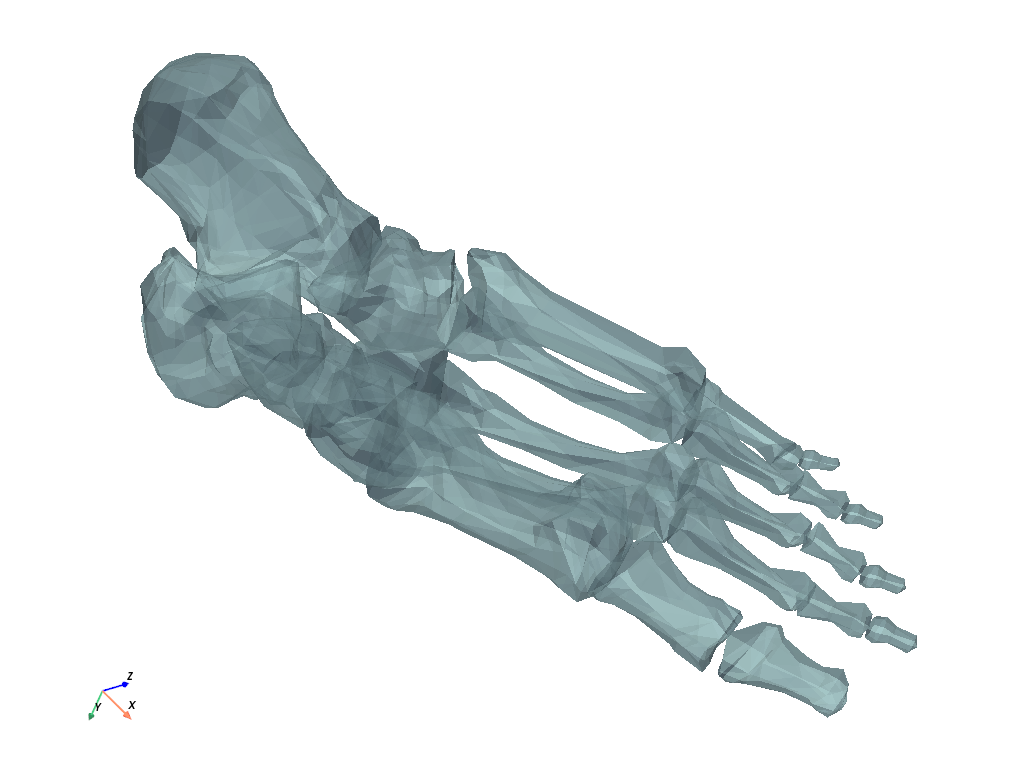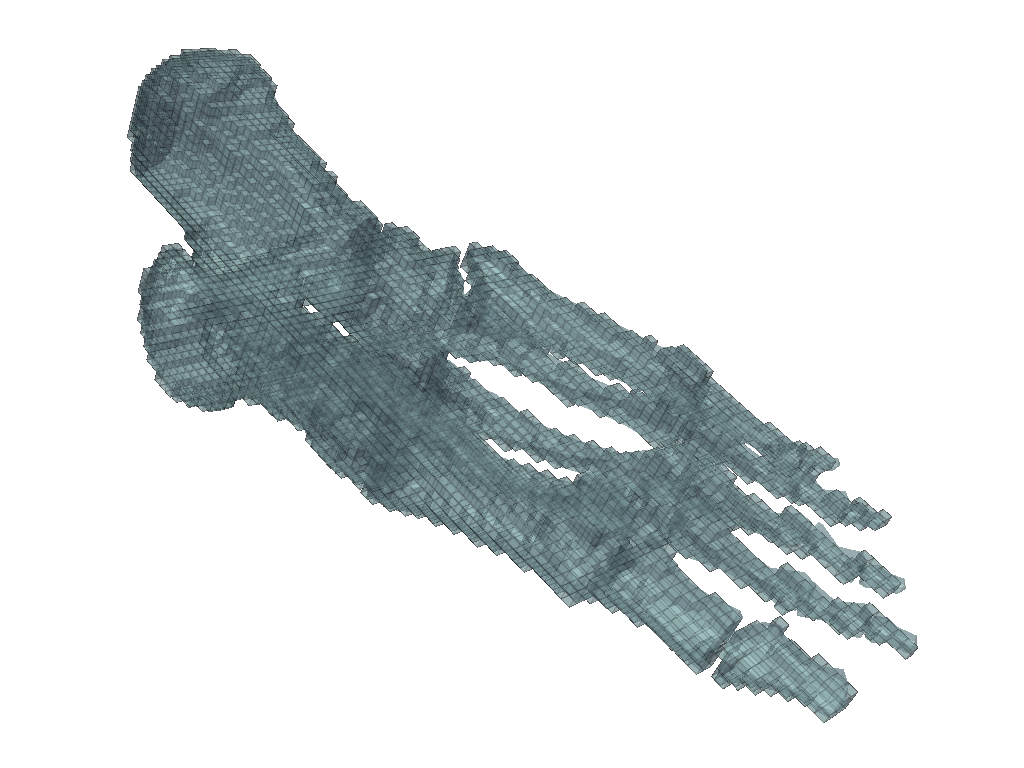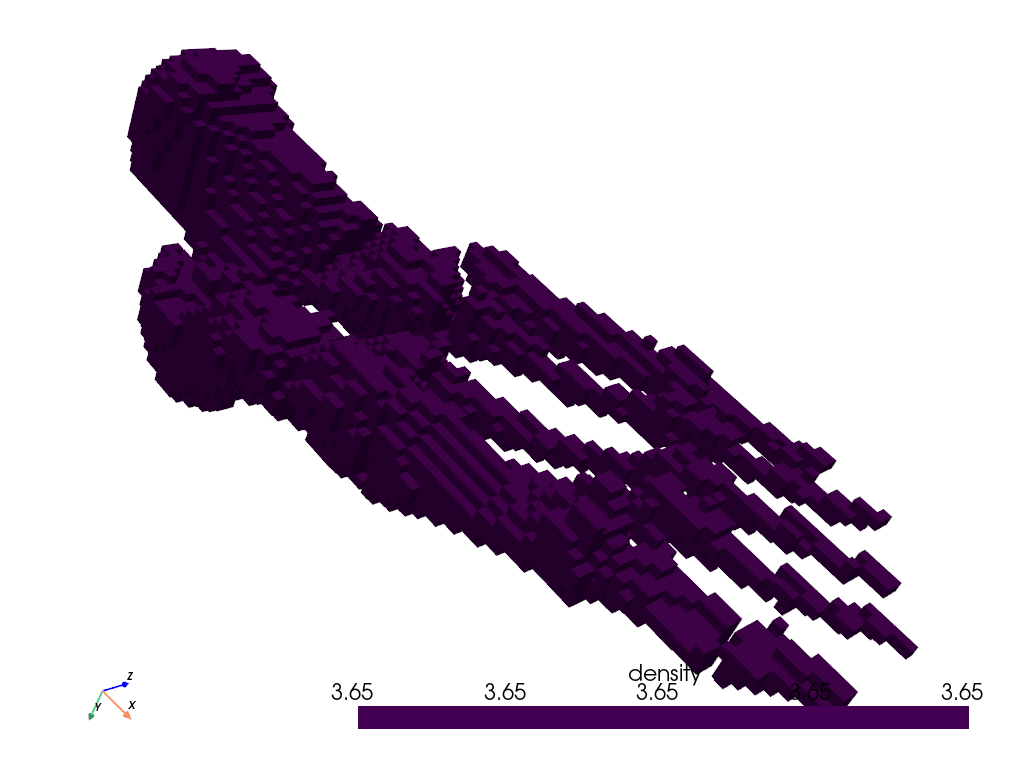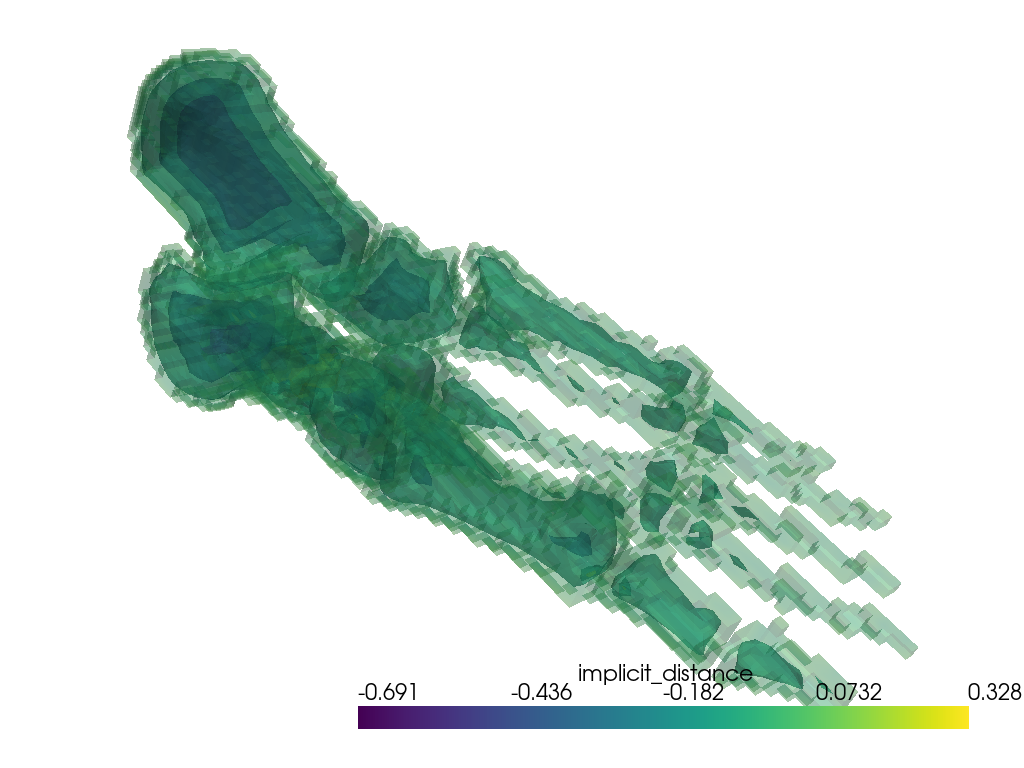# Voxelize a Surface Mesh#

Create a voxel model (like legos) of a closed surface or volumetric mesh.

This example also demonstrates how to compute an implicit distance from a bounding `pyvista.PolyData` surface.

```import numpy as np

import pyvista as pv

from pyvista import examples

# Load a surface to voxelize
surface
```
PolyDataInformation
N Cells4204
N Points2154
X Bounds-5.633e+00, 5.633e+00
Y Bounds-1.860e+00, 1.860e+00
Z Bounds-2.125e+00, 2.126e+00
N Arrays0

```cpos = [
(7.656346967151718, -9.802071079151158, -11.021236183314311),
(0.2224512272564101, -0.4594554282112895, 0.5549738359311297),
(-0.6279216753504941, -0.7513057097368635, 0.20311105371647392),
]

surface.plot(cpos=cpos, opacity=0.75)
```Create a voxel model of the bounding surface

```voxels = pv.voxelize(surface, density=surface.length / 200)

p = pv.Plotter()
p.add_mesh(voxels, color=True, show_edges=True, opacity=0.5)
p.show(cpos=cpos)
```We could even add a scalar field to that new voxel model in case we wanted to create grids for modelling. In this case, let’s add a scalar field for bone density noting:

```voxels["density"] = np.full(voxels.n_cells, 3.65)  # g/cc
voxels
```
UnstructuredGridInformation
N Cells93041
N Points113192
X Bounds-5.633e+00, 5.584e+00
Y Bounds-1.860e+00, 1.858e+00
Z Bounds-2.125e+00, 2.097e+00
N Arrays3
NameFieldTypeN CompMinMax
vtkOriginalPointIdsPointsint6413.685e+037.283e+05
vtkOriginalCellIdsCellsint6413.624e+037.017e+05
densityCellsfloat6413.650e+003.650e+00

```voxels.plot(scalars="density", cpos=cpos)
```A constant scalar field is kind of boring, so let’s get a little fancier by added a scalar field that varies by the distance from the bounding surface.

```voxels.compute_implicit_distance(surface, inplace=True)
voxels
```
UnstructuredGridInformation
N Cells93041
N Points113192
X Bounds-5.633e+00, 5.584e+00
Y Bounds-1.860e+00, 1.858e+00
Z Bounds-2.125e+00, 2.097e+00
N Arrays4
NameFieldTypeN CompMinMax
vtkOriginalPointIdsPointsint6413.685e+037.283e+05
implicit_distancePointsfloat641-6.951e-014.148e-01
vtkOriginalCellIdsCellsint6413.624e+037.017e+05
densityCellsfloat6413.650e+003.650e+00

```contours = voxels.contour(6, scalars="implicit_distance")

p = pv.Plotter()